Biomass Installation Feasibility ToolBoiler Sizing Manual

Knowing the energy demand of the building, one may size the boiler. As mentioned before, the boiler sizing process is based not on the peak demand, but on the mean load. Below, there is a screen shot of the main inputs that the user needs to provide: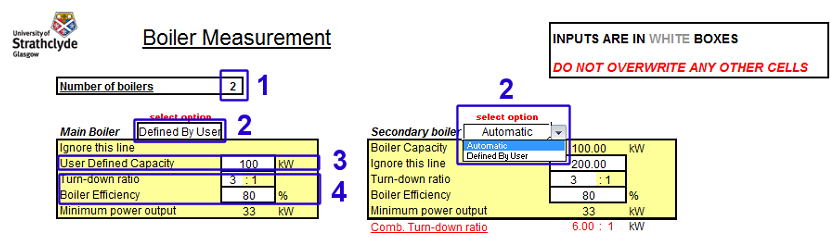# Inputs

1 – Number of boilers: In some cases the user may want to work with more than one biomass boiler at the same time. Various reasons may lead to that, for example rising the overall turndown ratio of the building until or in case of a site under construction, a phasing scheme for the heating circuit. On this screen the user selects between one or two boilers. In case the selection is one, the right table will be ignored and nothing written there will influence in the result.

2 – Boiler capacity defined by user: This menu gives the user an option of working with the size suggested by the tool or overwrite it with his/her own value. If working with two boilers, this option will be available for both of them, independently.

3 – User defined capacity: In this cell, the boiler capacity defined by the user is input. This value will just be used in the calculations if the option Defined by User is selected. This may be useful in case of sensitivity analysis, with a system where the boiler is already is working (so there is no interest on resizing it) or for systems with two boilers.

4 – Turn-down ratio and boiler efficiency: The user must know those values or leave the default ones (3:1 and 80%).

The inputs can be changed during any moment of the feasibility calculation, if it is necessary.

# The Outputs

## Boiler Size

In case the option Automatic is set, the tool will calculate the size of the boiler needed to supply the mean demand of the building, which was defined at the heat demand calculation part.

The screen presenting where those calculations will be displayed is shown below: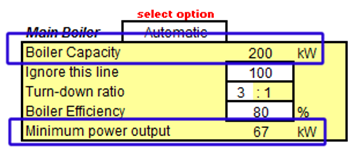To define the boiler capacity, a series of comparative algorithms were created. Initially the system will match the minimum output of the boiler with the mean load demand for the warmest month (1). After doing that and applying the turn down ratio it will observe where the maximum output will be located. For the reasons explained here the wood fuel boiler isn’t sized to supply the peak demand, just the monthly mean demand. If the boiler output calculated previously is higher than the mean demand during the coldest month (2b), the output will be set to the same value as this demand. A correction is applied to round the output to realistic value (for example if the calculation sizes a boiler of 53 kW, it will be corrected to 50 kW) and the minimum output will be also recalculated, defined by the turn-down ratio(3b). These steps are shown below, the dark blue line representing the mean load profile.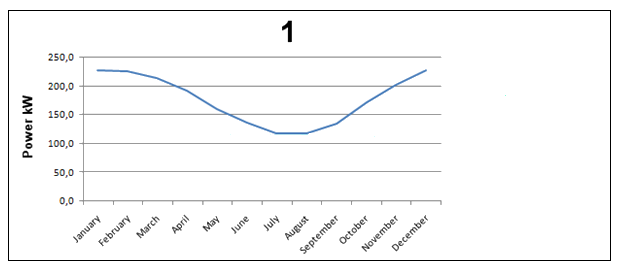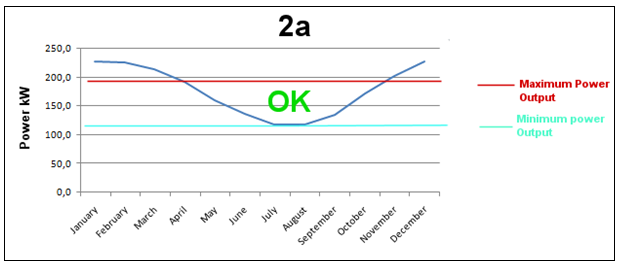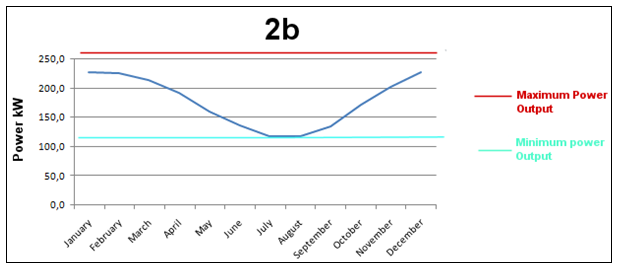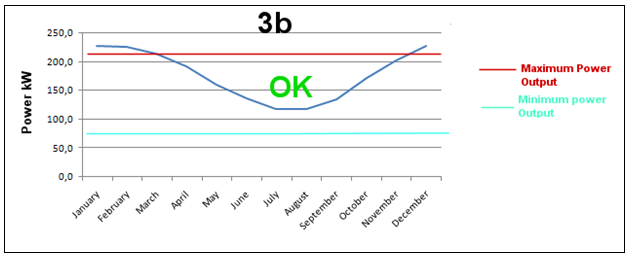After defining the size of the boiler, the following outputs are calculated: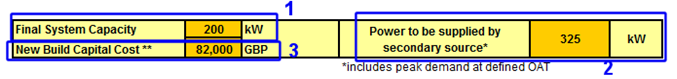1 – Final system capacity: Since there is a possibility to work with two boilers, it is important to have a cell where the power of both boilers is combined in order to define the final power capacity of the biomass system. It is done by simply adding the rated power of each boiler.

2 – Power to be supplied by a secondary source: As mentioned here, biomass systems in most cases requests a secondary source of power to back up or complement the demand not achieved by them. This calculation uses the maxim capacity of the boiler and compares it with the peak demand in the coldest month (calculated with the heat demand model). The difference is the power that needs to be supplied by a secondary source.

3 – New Build Capital Cost: In a feasibility study, as important as knowing the heating capacity of the boiler is to know how much it will cost. Using figures from a carbon trust report three range of costs were taken as shown in the table below:

 Power Output Base Cost Between 10 MW and 5 MW 360 GBP per kW Between 5MW and 300 kW 225 GBP per kW Under 300 kW 410 GBP per kW

These figures include the price of the boiler, installation and basic pipe work.

## Monthly Energy Consumption

Another very important calculation performed is the monthly energy consumption. Knowing the efficiency of the system it can be done easily by dividing the energy demand of the building by the boiler efficiency. Those numbers provide important information that will have direct impact on how much will be spent on fuel and the storage size required.